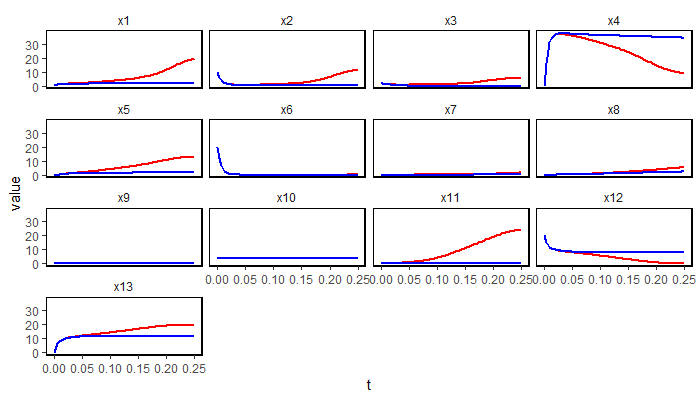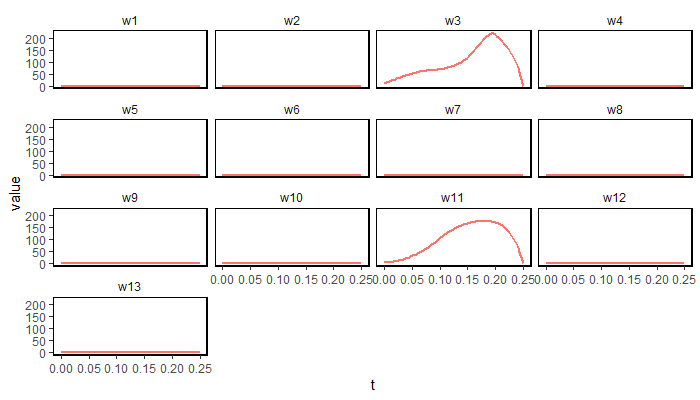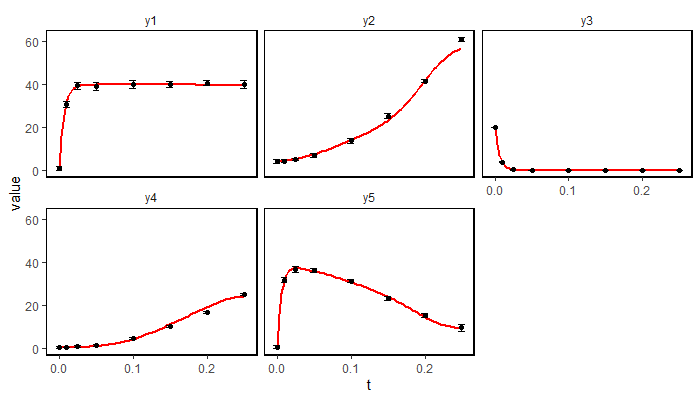# Introduction

The algorithms contained in the seeds package are implementation of the dynamic elastic net approach. There are two algorithms implemented that aim to calculate missing interactions between states of an ode in order to explain and minimize a systematic error within the model-equation. The hidden inputs should be an hint as to which states are missing interactions.

Both algorithm makes heavy use of the deSolve package. The use of Rtools is recommended to significantly shorten the computing time of the algorithm. It is not possible to use the BDEN algorithm without Rtools.

## The DEN (dynamic elastic net) Algorithm

The first Algorithm is a greedy method for generating a sparse solution for the hidden inputs. The algorithm is based on Pontryagin’s Maximum Principle and implements an iterative gradient descent method which aims at minimizing the Hamilton function of the system.

Rtools is suggested, because of the immense computational cost of the step size estimation of the gradient step.

## The odeModel Class

The class aims to be used to describe the experiment you want to analyze. It has the following slots:

• func: A function containing the ode-equations of the model. For syntax look at the given examples of the deSolve package.
• times: time steps at which the model should be evaluated
• parms: the parameters of the model
• input: matrix containing the inputs with the time points
• measFunc: function that converts the output of the ode solution
• y: initial (state) values of the ODE system, has to be a vector
• meas: matrix with the (experimental) measurements of the system
• sd: optional standard deviations of the measurements, is used by the algorithms as weights in the cost function
• custom: customized link function (is internally used)
• nnStates: bit vector that indicates if states should be observed by the root function
• nnTollerance: tolerance at which a function is seen as zero (optional)
• resetValue: value a state should be set to by an event (optional)

The class also provides methods to simply evaluate and display the nominal solution of an created odeModel object with the nominalSol and plot() function.

## Example

The following example uses the UVB protein g model and some simulated measurement data. An synthetic error has been added to the states $$x_3$$ and $$x_{11}$$.

The states of the model have to be written with the letter $$x$$ following by a number representing the state. If an input is needed for the model the input takes the letter $$u$$. This restrictions are made to ensure that the automatic differentiation will work as intended.

library(seeds)

# usb network example
uvbParameter = c(  ks1=0.23,
ks2=4.0526,
kdr1=0.1,
kdr2=0.2118,
k1=0.0043,
k2=161.62,
ka1=0.0372,
ka2=0.0611,
ka3=4.7207,
kd1=94.3524,
kd2=50.6973,
kd3=0.5508,
ks3=0.4397,
kdr3=1.246,
uv=1,
ka4=10.1285,
kd4=1.1999,
n1=3,
n2=2,
n3=3.5,
kdr3a=0.9735,
kdr3b=0.406,
ksr=0.7537,
fhy3_s=5)
# initial state
x0 = c(0.2,10,2,0,0,20,0,0,0,4.2,0.25,20,0)

# model ode
uvbModel <- function(t,x,parameters) {
with (as.list(parameters),{

dx1 = ((-2) * ((ka1 * (x^2) * (x^2)) - (kd1 * x)) +
(-2) * ((ka2 * (x^2) * x) - (kd2 * x)) +
((ks1 *((1) + (uv * n3 * (x + fhy3_s))))  -
(kdr1 * ((1) + (n1 * uv)) * x)))
dx2 = ((-1) * ((ka2*(x^2) * x) - (kd2 * x)) +
(-1) * ((ka4 * x * x) - (kd4 * x)))
dx3 = (((ka2 * (x^2) * x) - (kd2*  x)))
dx4 = ((-2) * (k1*(x^2)) + (2) * (k2 * x) +
(-2) * ((ka1 * (x^2)* (x^2)) - (kd1 * x)) +
(-1)* (ka3 * x *x))
dx5 =  (((ka1 * (x^2) * (x^2)) -(kd1 * x)))
dx6 = ((-1) * (k2 * x) +  (k1 * (x^2)) +(kd3 * (x^2)))
dx7 = ((-1) * (ka3 * x * x) + ((ks2 * ((1) + (uv * x))) -
(kdr2 * x)) + (2) * (kd3 * (x^2)))
dx8 = ((-2) * (kd3 * x^2) + (ka3 * x * x))
dx9  = 0
dx10 = 0
dx11 =  (((ks3 * ((1) + (n2 * uv))) -(kdr3 * (((x / (kdr3a + x)) +
(x / (kdr3b + x))) -(x / (ksr + x))) *  x)))
dx12 = ((-1) * (ka4 * x * x) + (kd4 * x))
dx13 =((ka4 * x * x) - (kd4 * x))

list(c(dx1,dx2,dx3,dx4,dx5,dx6,dx7,dx8,dx9,dx10,dx11,dx12,dx13))
})
}

# measurement function
uvbMeasure <- function(x) {

y1 = 2*x[,5] + x[,4] + x[,8]
y2 = 2*x[,5] + 2* x[,3] + x[,1]
y3 = x[,6]
y4 = x[,11]
y5 = x[,4]

return(cbind(y1,y2,y3,y4,y5))
}

# measurement data
y <- uvbData[,1:6]
# timesteps
t <- uvbData$t # standard deviation of the measurements sd <- uvbData[,7:11] uvbModel <- odeModel(func = uvbModel, parms = uvbParameter, times = t, measFunc = uvbMeasure, y = x0, meas = y, sd = sd) res <- DEN(odeModel = uvbModel, alphaStep = 500, alpha2 = 0.0001, epsilon = 0.2, plotEstimates = FALSE) The results are returned as list of resultsSeeds objects, which store the estimated states, measurements and the hidden inputs of a model. A standard plot function is given to display the results. Functions for annotating the plots are also included in the package. The use of the BDEN algorithm displays not only the calculated states and inputs, but also confidence intervals for the corresponding states of the system. ### Plotting The standard plot gives you three figures: 1. The estimated states (blue without hidden inputs, red with hidden inputs) 2. The estimated hidden inputs 3. The estimated measurements. (blue without hidden inputs, red with hidden inputs) All calculated values can be output by methods of the class odeModel, or extracted directly by the corresponding slots. The structure and methods of the class can be found in the corresponding documentation. # plot the solution of the second iteration plot(res[]) The plotting function will give you three plots.Estimated States for the modelEstimated hidden inputsMeasurements resulting from the estimated hidden inputs # Bayesian Dynamic Elastic Net Full Bayesian algorithm to detect hidden inputs in ODE based models.The algorithm is an extension of the Dynamic Elastic Net algorithm (Engelhardt et al. 2016) inspired by the Elastic-Net Regression. Ordinary differential equations (ODEs) are a popular approach to quantitatively model molecular networks based on biological knowledge. However, such knowledge is typically restricted. Wrongly modeled biological mechanisms as well as relevant external influence factors that are not included into the model likely manifest in major discrepancies between model predictions and experimental data. Finding the exact reasons for such observed discrepancies can be quite challenging in practice. In order to address this issue we suggest a Bayesian approach to estimate hidden influences in ODE based models. The method can distinguish between exogenous and endogenous hidden influences. Thus, we can detect wrongly specified as well as missed molecular interactions in the model. The BDEN as a new and fully probabilistic approach, supports the modeler in an algorithmic manner to identify possible sources of errors in ODE based models on the basis of experimental data. THE BDEN does not require pre-specified hyper-parameters. BDEN thus provides a systematic Bayesian computational method to identify target nodes and reconstruct the corresponding error signal including detection of missing and wrong molecular interactions within the assumed model. The method works for ODE based systems even with uncertain knowledge and noisy data. In contrast to approaches based on point estimates the Bayesian framework incorporates the given uncertainty and circumvents numerical pitfalls which frequently arise from optimization methods (Engelhardt et al. 2017). ## BDEN The algorithm can be executed in a similar fashion as the DEN algorithm. However computational time is much higher. See demonstration on how to use the algorithm. For further information look into the documentation of the functions. library(seeds) uvbParameter = c( ks1=0.23, ks2=4.0526, kdr1=0.1, kdr2=0.2118, k1=0.0043, k2=161.62, ka1=0.0372, ka2=0.0611, ka3=4.7207, kd1=94.3524, kd2=50.6973, kd3=0.5508, ks3=0.4397, kdr3=1.246, uv=1, ka4=10.1285, kd4=1.1999, n1=3, n2=2, n3=3.5, kdr3a=0.9735, kdr3b=0.406, ksr=0.7537, fhy3_s=5) x0 = c(0.2,10,2,0,0,20,0,0,0,4.2,0.25,20,0)+0.00001 uvbModel <- function(t,x,parameters) { with (as.list(parameters),{ dx1 = ((-2) * ((ka1 * (x^2) * (x^2)) - (kd1 * x)) + (-2) * ((ka2 * (x^2) * x) - (kd2 * x)) + ((ks1 *((1) + (uv * n3 * (x + fhy3_s)))) - (kdr1 * ((1) + (n1 * uv)) * x))) dx2 = ((-1) * ((ka2*(x^2) * x) - (kd2 * x)) + (-1) * ((ka4 * x * x) - (kd4 * x))) dx3 = (((ka2 * (x^2) * x) - (kd2* x))) dx4 = ((-2) * (k1*(x^2)) + (2) * (k2 * x) + (-2) * ((ka1 * (x^2)* (x^2)) - (kd1 * x)) + (-1)* (ka3 * x *x)) dx5 = (((ka1 * (x^2) * (x^2)) -(kd1 * x))) dx6 = ((-1) * (k2 * x) + (k1 * (x^2)) +(kd3 * (x^2))) dx7 = ((-1) * (ka3 * x * x) + ((ks2 * ((1) + (uv * x))) - (kdr2 * x)) + (2) * (kd3 * (x^2))) dx8 = ((-2) * (kd3 * x^2) + (ka3 * x * x)) dx9 = 0 dx10 = 0 dx11 = (((ks3 * ((1) + (n2 * uv))) -(kdr3 * (((x / (kdr3a + x)) + (x / (kdr3b + x))) -(x / (ksr + x))) * x))) dx12 = ((-1) * (ka4 * x * x) + (kd4 * x)) dx13 =((ka4 * x * x) - (kd4 * x)) list(c(dx1,dx2,dx3,dx4,dx5,dx6,dx7,dx8,dx9,dx10,dx11,dx12,dx13)) }) } uvbMeasure <- function(y,parameter){ y1 = 2*y[,5] + y[,4] + y[,8] y2 = 2*y[,5] + 2* y[,3] + y[,1] y3 = y[,6] y4 = y[,11] y5 = y[,4] return(cbind(y1,y2,y3,y4,y5)) } testNN = rep(0,length(x0)) testNN = 1 y <- uvbData[,1:6] t <- uvbData$t
sd <- uvbData[,7:11]

Model <- odeModel(func = uvbModel, parms = uvbParameter,times=c(0.025),
measFunc = uvbMeasure, y = x0, meas = y, sd = sd,custom=TRUE)

A <- BDEN(odeModel          = Model,
lambda            = .001,
beta_init         = c(1,1,1,1,1),
numbertrialsstep  = 15,
numbertrialseps   = 2000,
numbertrialinner  = 10)